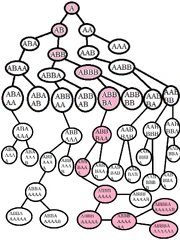10,954 PagesNotes: Tree shows only acceptable sequences inscribed in each node; Inverse sequences (where A's replaced by B's and vice versa) aren't shown; Painted purple nodes are members of the longest sequence.

The block subsequence theorem is a theorem due to Harvey Friedman. It shows that a string with a certain property involving subsequences cannot be infinite. The length of the largest string possible is a function of the alphabet size — the numbers that it generates immediately grow very large. Two numbers in the sequence, n(3) and n(4), are the subject of extensive research.

Suppose we have a string x1, x2, ... (We call such a string a Friedman string.) made of an alphabet of k letters, such that no block of letters xi, ..., x2i is a substring of any later block xj, ..., x2j. Friedman showed that such a string cannot be infinite, and for every k there is a sequence of maximal length. Call this length n(k).

## Сomputation

n(k) is computable, and hence there exists an algorithm to calculate it:

1. Rewrite number with k-ary positional system and keep it.
2. Increase number by 1.
3. Check whether this number represents Friedman string.
4. If there is no number with m digits which represents Friedman string, then n(k)=m-1.

## Values

• \(n(1) = 3\), using the string "111". "1111" does not satisfy the conditions since x1,x2 = "11" is a subsequence of x2,x3,x4 = "111".
• \(n(2) = 11\), using the strings "12221111111" or "12221111112". For example, "122211111121" doesn't satisfy the conditions because x1,x2="12" is a subsequence of x6,x7,...,x12="1111121".
• \(n(3)\) and \(n(4)\) are much larger. The strings which they used aren't known, but Friedman gave the string "1221317321313813513201235313108" = "122131111111331313333333313333313333333333333333333311..." with length 216 for \(n(3)\).
• Define a version of the Ackermann function as follows:
\(A(1, n) = 2n\)
\(A(k + 1, n) = 2 \uparrow^k n\)
\(A(n) = A(n, n)\)
• \(A(7\,198, 158\,386) < n(3) < A(A(5))\)
• \(n(4) > A^{A(187,196)}(1)\)

Friedman has demonstrated that the growth rate of the n function lies asymptotically between \(f_{ω^ω}(n)\) and \(f_{ω^ω+1}(n)\) in the fast-growing hierarchy. He has also stated that every n(k) is odd. Chris Bird notes that n(k) is "broadly comparable" to {3, 3, ..., 3, 3} (with k 3's) in Bowers' BEAF, or k & 3. For comparison, Graham's number is only about {3, 65, 1, 2}.

n(k) is related to the TREE sequence, which grows even faster.